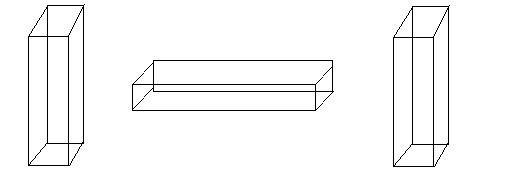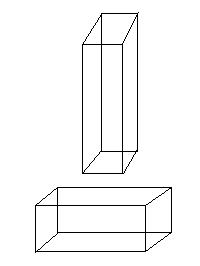Article

# Calculating the Weight of Stone

This is a guide intended to help anyone get the approximate weight of a stone without having to use a scale. The only required tools are a calculator, tape measure, pencil, and paper. Using the above tools allows the user to safely calculate weight prior to lifting stone with a hoist to prevent damage to the equipment or the people operating it.

## Step 1: Calculating the cubic feet of the stone

The most important part of step one is to measure correctly. In this guide we will be measuring in feet. The materials needed for step one include the tape measure, pencil, and paper. You need to measure the length, width and height of the stone you plan on weighing. Then you multiply the length width and the height together to get the stone’s cubic feet. Since it is best to have a higher cubic feet calculation than a lower one to ensure your equipment will be able to handle the load, you can round up. We will round up all the measurements for the rest of this guide.

For example: In the case when the stone has an arc located at the top, measure the height perpendicular to the top of the arc. We can then multiply the length, width, and the height together and obtain the cubic feet.

Sometimes you might have to measure the stone in parts and then add the cubic feet of the separate parts together.Parts of a cross at the top of a monument. If we were to break the cross in two the middle would be measured twice, therefore we break it into three parts. National Park Service

For example: In this case we need to separate this monument into three parts and then add the cubic feet of each separate part together.

First we will start with the cross at the top of the monument. We will break this into three separate parts as shown in the picture below.

If we were to break this in two the middle would be measured twice, therefore we break it into three parts. Once you measure the length, width, and height of these pieces you multiply them together to calculate the cubic feet of each part of the cross, then add them together for the cubic feet of the whole cross.Block and base of stone grave monument. We will measure this one and add its cubic feet to that of the cross. National Park Service

The next part we will break up is the large block underneath the cross and above the base. We will measure this one and add its cubic feet to that of the cross. Now we have the cubic feet of the cross and large block.

The next part we will break up is the base of this monument. Again, we will measure the length, width, and height and add the cubic feet to that of the rest of the monument and obtain the cubic feet of the entire monument.

Now that we have gone through the way to calculate the cubic feet of the stone you are working with we can move on to step 2.Crosses atop pedestal grave markers at Oakdale Cemetery, Wilimington, NC. National Park Service

## Step 2: Calculating the weight of the stone

In this step you will be using the cubic feet you obtained from doing step 1 to calculate the approximate weight of the stone. You will need a calculator, pencil, and paper to do this.

Now that you have your cubic feet written down all you need to do is use the chart below. First off, locate the type of stone you are using on the chart. Next multiply the cubic feet you obtained from using step one to the number in the minimum lbs/ft3 column. That number is approximately the minimum weight of the stone. You then do the same in the maximum lbs/ft3 column to obtain the maximum weight of the stone.

Calculating the minimum and maximum weight of stone
Type of Stone Minimum lbs./ft3 Maximum lbs./ft3
Marble 150 lbs./ft3 179 lbs./ft3
Granite 159 lbs./ft3 180 lbs./ft3
Limestone 150 lbs./ft3 179 lbs./ft3

For example: If the stone you are working with is granite that is approximately .64ft3 your calculation should look like the following; ft3 x lbs/ft3 = lbs so your calculation would be; minimum lbs= .64 x 159 = 101.8, maximum lbs = .64 x 180 = 115.1

Therefore the granite is between 101.8 and 115.1 pounds.

The weight is given in a range because not all stones of the same type weigh the same. Also, this accounts for carvings and the rounding done during step 1.

Now that you know the approximate weight of the stone you can use it to see if your equipment can handle the load. Note that this is calculated for a dry stone 10%-20% should be added for a stone if it has rained in the recent past.

So a stone weighing 500 lbs dry gets, damp may weigh upwards of 600 lbs.

## Resources

Civil Engineer’s Pocket-Book by John. C. Trautwine. 121st Thousand 19th Edition, Forth Issue. Trautwine Company.

## Tags

Last updated: September 27, 2021